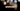# Leetcode | Solution of Number of Steps to Reduce a Number to Zero

March 21st, 2020
|

In this post, we will solve number of steps to reduce a number to zero from leetcode and compute the time and space complexities. Let's begin.

# Problem Statement

The question can be found at leetcode number of steps to reduce a number to zero problem.

The problem states that we need to determine how many steps would it take to reduce a number to zero based on given constraints

# Constraints and challenges

The following operations should be applied to the number

• if the number is even, divide it by 2
• if it's odd, subtract it by 1

# Solution

We will discuss a solution using simple while loop

The question is pretty simple and logic is as staright forward as it can get

• we will declare a counter and assign it a value zero
• we'll run a while loop until the number is zero
• in the loop, we'll check if the number if even or odd
• respectively, we'll either divide it by 2 or subtract by 1
• we'll increase the counter by 1 in each operation
• once the number is zero, we'll jump out of loop and return our counter

We have discussed the approach, I urge you to go ahead on leetcode and give it another try.If you are here, it means something went wrong in implementation or you are just too lazy. In any case, let's see a simple implementation of the above logic.
``````var numberOfSteps = function (num) {
let count = 0;

while (num > 0) {
if (num % 2 === 0) {
num = num / 2;
} else {
num -= 1;
}
count++;
}

return count;
};``````

The solution is very straight forward. It is just the code counterpart of the logic we discussed above.

``````Status: Accepted
Runtime: 56ms
Memory: 33.7MB``````

## Time and space complexity

### Time complexity

We are looping over the number and decreasing it in half every second time, so time complexity would be O(log n).

### Space complexity

We are not using any extra space except for a couple of variables. So the space complexity is constant, O(1).

# Summary

So, we solved the number of steps to reduce a number to zero problem by using a simple while loop and calculated the time and space complexities.

I hope you enjoyed solving this question. This is it for this one, complete source code for this post can be found on my Github Repo. Will see you in the next one.